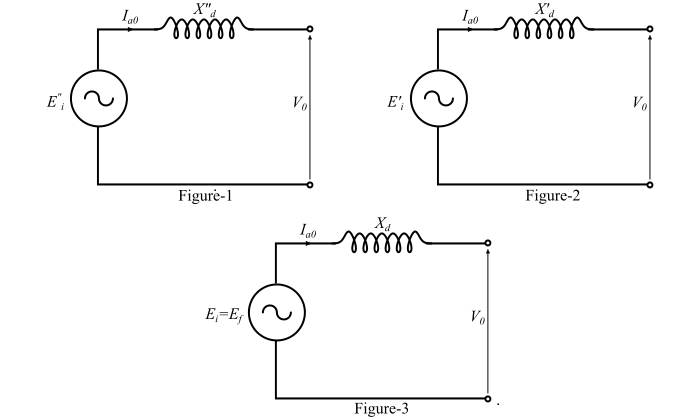# Sudden Short Circuit of Three-Phase Alternator

Short circuits at the terminals of the unloaded alternators or synchronous generators are very rare. They generally occur due to insulation failure or accidental damage on some part of the power system supplied by the generator. Therefore, it is important to deal with the case of a 3-phase alternator delivering power to a load or to an infinite bus.

If a short circuit occurs across the armature terminals of the alternator, the short circuit armature current will pass through a sub-transient period, a transient period and finally will settle down to a steady-state condition. Also, when the short circuit occurs, the reactance of the machine changes from $𝑋_{𝑑}$ to $𝑋^{"}_{𝑑}$ . In order to satisfy the initial conditions of constant flux linkage, the excitation voltages must also change.

Figures 1, 2 and 3 show the equivalent circuits during sub-transient, transient and steady-state periods, respectively.Refer the figures, the voltages $𝐸_{𝑖}, 𝐸^{′}_{𝑖}$ and $𝐸^{"}_{𝑖}$ and are the internal voltages and they are determined from the prefault conditions. If $𝑉_{0}$ is the terminal voltage of the machine and $𝐼_{𝑎0}$ is the prefault steady-state armature current, then

• The voltage behind sub-transient reactance before fault is,

$$\mathrm{{𝐸^{"}_{𝑖}} = 𝑉_{0} + 𝑗𝐼_{𝑎0}{𝑋^{"}_{𝑑}} … (1)}$$

• The voltage behind transient reactance before fault is,

$$\mathrm{{𝐸^{′}_{𝑖}} = 𝑉_{0} + 𝑗𝐼_{𝑎0}{𝑋^{′}_{𝑑}} … (2)}$$

• The voltage behind synchronous reactance before fault is,

$$\mathrm{𝐸_{𝑖} = 𝐸_{𝑓} = 𝑉_{0} + 𝑗𝐼_{𝑎0}𝑋_{𝑑} … (3)}$$

Therefore, the sub-transient current during short-circuit is,

$$\mathrm{𝐼^{"} =\frac{𝐸^{"}_{𝑖}}{𝑋^{"}_{𝑑}}… (4)}$$

The transient current during short circuit is,

$$\mathrm{𝐼^{′} =\frac{𝐸^{′}_{𝑖}}{𝑋^{′}_{𝑑}}… (5)}$$

The steady-state short circuit current is,

$$\mathrm{𝐼_{𝑠𝑠} =\frac{𝐸_{𝑖}}{𝑋_{𝑑}}=\frac{𝐸_{𝑓}}{𝑋_{𝑑}}… (6)}$$

Hence, the short-circuit current of the machine is given by,

$$\mathrm{𝐼_{𝑠𝑐}(𝑡) = (𝐼^{"} − 𝐼^{′})𝑒^{−𝑡⁄𝜏^{"}_{d}} + (𝐼^{′} − 𝐼_{𝑠𝑠})𝑒^{−𝑡⁄𝜏^{'}_{d}} + 𝐼_{𝑠𝑠}}$$

$$\mathrm{\Rightarrow\:𝐼_{𝑠𝑐}(𝑡) =\left(\frac{𝐸^{"}_{𝑖}}{𝑋^{"}_{𝑑}}-\frac{𝐸^{′}_{𝑖}}{𝑋^{′}_{𝑑}}\right)𝑒^{−𝑡⁄𝜏^{"}_{d}}+ \left(\frac{𝐸^{′}_{𝑖}}{𝑋^{′}_{𝑑}}- \frac{𝐸_{𝑖}}{𝑋_{𝑑}}\right)𝑒^{−𝑡⁄𝜏^{'}_{d}}+\frac{𝐸_{𝑖}}{𝑋_{𝑑}}… (7)}$$

Where,

$$\mathrm{{𝜏^{′}_{𝑑}} = \frac{𝑋^{'}_{𝑑}}{𝑋_{𝑑}}{𝜏^{′}_{𝑑0}}}$$

$$\mathrm{{𝜏^{"}_{𝑑}} = \frac{𝑋^{"}_{𝑑}}{𝑋^{′}_{𝑑}}{𝜏^{"}_{𝑑0}}}$$